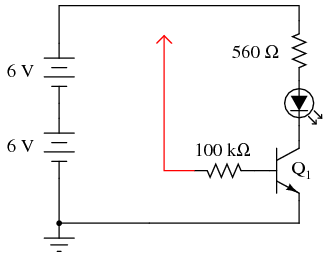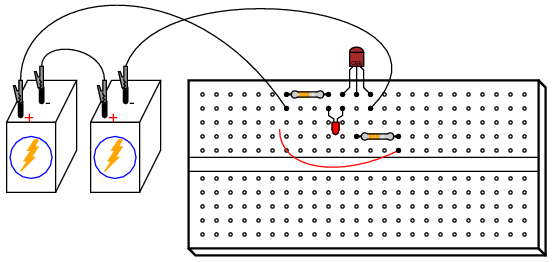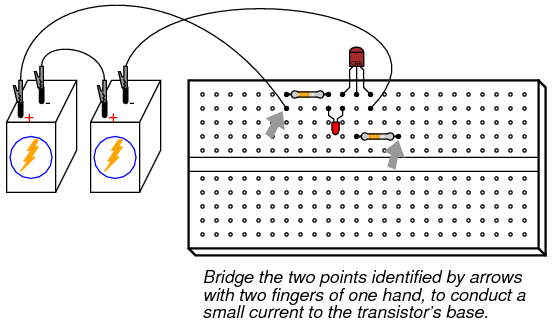# 5.8: Transistor as a Switch

$$\newcommand{\vecs}{\overset { \rightharpoonup} {\mathbf{#1}} }$$ $$\newcommand{\vecd}{\overset{-\!-\!\rightharpoonup}{\vphantom{a}\smash {#1}}}$$$$\newcommand{\id}{\mathrm{id}}$$ $$\newcommand{\Span}{\mathrm{span}}$$ $$\newcommand{\kernel}{\mathrm{null}\,}$$ $$\newcommand{\range}{\mathrm{range}\,}$$ $$\newcommand{\RealPart}{\mathrm{Re}}$$ $$\newcommand{\ImaginaryPart}{\mathrm{Im}}$$ $$\newcommand{\Argument}{\mathrm{Arg}}$$ $$\newcommand{\norm}{\| #1 \|}$$ $$\newcommand{\inner}{\langle #1, #2 \rangle}$$ $$\newcommand{\Span}{\mathrm{span}}$$ $$\newcommand{\id}{\mathrm{id}}$$ $$\newcommand{\Span}{\mathrm{span}}$$ $$\newcommand{\kernel}{\mathrm{null}\,}$$ $$\newcommand{\range}{\mathrm{range}\,}$$ $$\newcommand{\RealPart}{\mathrm{Re}}$$ $$\newcommand{\ImaginaryPart}{\mathrm{Im}}$$ $$\newcommand{\Argument}{\mathrm{Arg}}$$ $$\newcommand{\norm}{\| #1 \|}$$ $$\newcommand{\inner}{\langle #1, #2 \rangle}$$ $$\newcommand{\Span}{\mathrm{span}}$$$$\newcommand{\AA}{\unicode[.8,0]{x212B}}$$

PARTS AND MATERIALS

• Two 6-volt batteries
• One NPN transistor—models 2N2222 or 2N3403 recommended (Radio Shack catalog # 276-1617 is a package of fifteen NPN transistors ideal for this and other experiments)
• One 100 kΩ resistor
• One 560 Ω resistor
• One light-emitting diode (Radio Shack catalog # 276-026 or equivalent)

Resistor values are not critical for this experiment. Neither is the particular light emitting diode (LED) selected.

CROSS-REFERENCES

Lessons In Electric Circuits, Volume 3, chapter 4: “Bipolar Junction Transistors”

LEARNING OBJECTIVES

• Current amplification of a bipolar junction transistor

SCHEMATIC DIAGRAMILLUSTRATIONINSTRUCTIONS

The red wire shown in the diagram (the one terminating in an arrowhead, connected to one end of the 100 kΩ resistor) is intended to remain loose, so that you may touch it momentarily to other points in the circuit.resistor) is intended to remain loose, so that you may touch it momentarily to other points in the circuit.

If you touch the end of the loose wire to any point in the circuit more positive than it, such as the positive side of the DC power source, the LED should light up. It takes 20 mA to fully illuminate a standard LED, so this behavior should strike you as interesting because the 100 kΩ resistor to which the loose wire is attached restricts current through it to a far lesser value than 20 mA. At most, a total voltage of 12 volts across a 100 kΩ resistance yields a current of only 0.12 mA, or 120 µA! The connection made by your touching the wire to a positive point in the circuit conducts far less current than 1 mA, yet through the amplifying action of the transistor, is able to control a much greater current through the LED.

Try using an ammeter to connect the loose wire to the positive side of the power source, like this:You may have to select the most sensitive current range on the meter to measure this small flow. After measuring this controlling current, try measuring the LED’s current (the controlled current) and compare magnitudes. Don’t be surprised if you find a ratio in excess of 200 (the controlled current 200 times as great as the controlling current)!

As you can see, the transistor is acting as a kind of electrically-controlled switch, switching current on and off to the LED at the command of a much smaller current signal conducted through its base terminal.

To further illustrate just how minuscule the controlling current is, remove the loose wire from the circuit and try “bridging” the unconnected end of the 100 kΩ resistor to the power source’s positive pole with two fingers of one hand. You may need to wet the ends of those fingers to maximize conductivity:Try varying the contact pressure of your fingers with these two points in the circuit to vary the amount of resistance in the controlling current’s path. Can you vary the brightness of the LED by doing so? What does this indicate about the transistor’s ability to act as more than just a switch; i.e. as a variable

COMPUTER SIMULATION

Schematic with SPICE node numbers:Netlist (make a text file containing the following text, verbatim):In this simulation, the voltage drop across the 560 Ω resistor v(1,3) turns out to be 10.26 volts, indicating a LED current of 18.32 mA by Ohm’s Law (I=E/R). R1‘s voltage drop (voltage between nodes 1 and 2) ends up being 11.15 volts, which across 100 kΩ gives a current of only 111.5 µA. Obviously, a very small current is exerting control over a much larger current in this circuit.

In case you were wondering, the is=1e-28 parameter in the diode’s .model line is there to make the diode act more like an LED with a higher forward voltage drop.

This page titled 5.8: Transistor as a Switch is shared under a GNU Free Documentation License 1.3 license and was authored, remixed, and/or curated by Tony R. Kuphaldt (All About Circuits) via source content that was edited to the style and standards of the LibreTexts platform; a detailed edit history is available upon request.